# Python | Pandas Merging, Joining, and Concatenating

• Last Updated : 14 Jan, 2019

Pandas DataFrame is two-dimensional size-mutable, potentially heterogeneous tabular data structure with labelled axes (rows and columns). A Data frame is a two-dimensional data structure, i.e., data is aligned in a tabular fashion in rows and columns. We can join, merge, and concat dataframe using different methods. In Dataframe `df.merge()`,`df.join()`, and `df.concat()` methods help in joining, merging and concating different dataframe.#### Concatenating DataFrame

In order to concat dataframe, we use `concat()` function which helps in concatenating a dataframe. We can concat a dataframe in many different ways, they are:

• Concatenating DataFrame using `.concat()`
• Concatenating DataFrame by setting logic on axes
• Concatenating DataFrame using `.append()`
• Concatenating DataFrame by ignoring indexes
• Concatenating DataFrame with group keys
• Concatenating with mixed ndims

Concatenating DataFrame using `.concat()` :
In order to concat a dataframe, we use `.concat()` function this function concat a dataframe and returns a new dataframe.

```# importing pandas module
import pandas as pd

# Define a dictionary containing employee data
data1 = {'Name':['Jai', 'Princi', 'Gaurav', 'Anuj'],
'Age':[27, 24, 22, 32],
'Qualification':['Msc', 'MA', 'MCA', 'Phd']}

# Define a dictionary containing employee data
data2 = {'Name':['Abhi', 'Ayushi', 'Dhiraj', 'Hitesh'],
'Age':[17, 14, 12, 52],
'Qualification':['Btech', 'B.A', 'Bcom', 'B.hons']}

# Convert the dictionary into DataFrame
df = pd.DataFrame(data1,index=[0, 1, 2, 3])

# Convert the dictionary into DataFrame
df1 = pd.DataFrame(data2, index=[4, 5, 6, 7])

print(df, "\n\n", df1)

```

Now we apply `.concat` function in order to concat two dataframe

```# using a .concat() method
frames = [df, df1]

res1 = pd.concat(frames)
res1
```

Output :
As shown in the output image, we have created two dataframe after concatenating we get one dataframe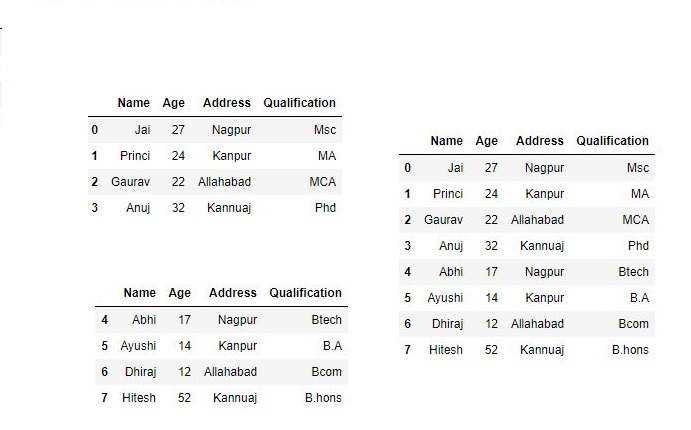Concatenating DataFrame by setting logic on axes :
In order to concat dataframe, we have to set different logic on axes. We can set axes in the following three ways:

• Taking the union of them all, `join='outer'`. This is the default option as it results in zero information loss.
• Taking the intersection, `join='inner'`.
• Use a specific index, as passed to the `join_axes` argument
```# importing pandas module
import pandas as pd

# Define a dictionary containing employee data
data1 = {'Name':['Jai', 'Princi', 'Gaurav', 'Anuj'],
'Age':[27, 24, 22, 32],
'Qualification':['Msc', 'MA', 'MCA', 'Phd'],
'Mobile No': [97, 91, 58, 76]}

# Define a dictionary containing employee data
data2 = {'Name':['Gaurav', 'Anuj', 'Dhiraj', 'Hitesh'],
'Age':[22, 32, 12, 52],
'Qualification':['MCA', 'Phd', 'Bcom', 'B.hons'],
'Salary':[1000, 2000, 3000, 4000]}

# Convert the dictionary into DataFrame
df = pd.DataFrame(data1,index=[0, 1, 2, 3])

# Convert the dictionary into DataFrame
df1 = pd.DataFrame(data2, index=[2, 3, 6, 7])

print(df, "\n\n", df1)
```

Now we set axes `join = inner` for intersection of dataframe

```# applying concat with axes
# join = 'inner'
res2 = pd.concat([df, df1], axis=1, join='inner')

res2
```

Output :
As shown in the output image, we get the intersection of dataframe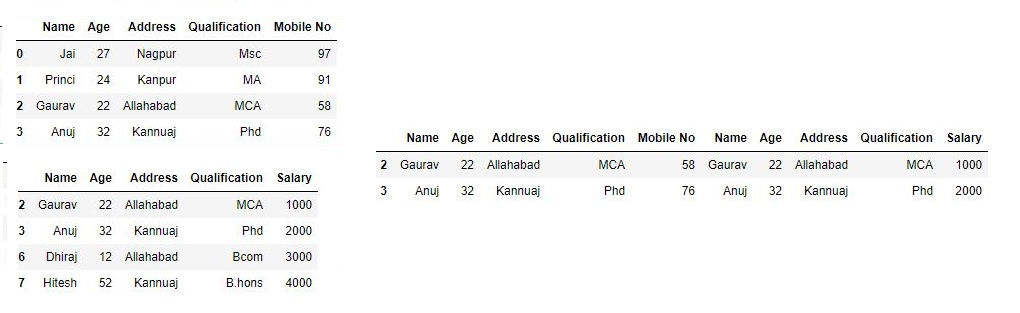Now we set axes `join = outer` for union of dataframe.

```# using a .concat for
# union of dataframe
res2 = pd.concat([df, df1], axis=1, sort=False)

res2
```

Output :
As shown in the output image, we get the union of dataframe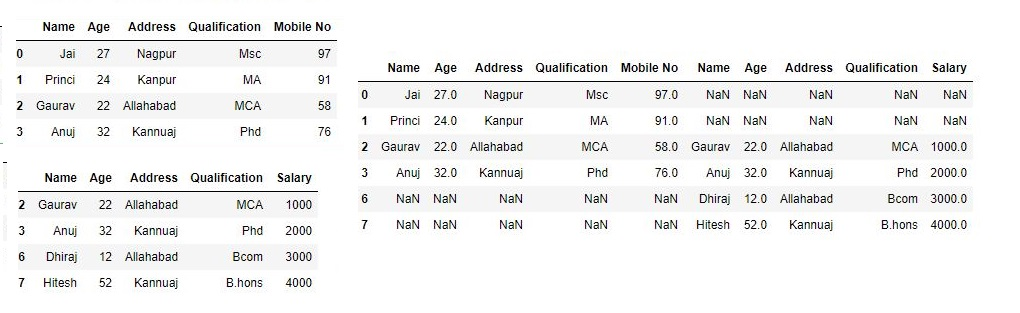Now we used a specific index, as passed to the `join_axes` argument

```# using join_axes
res3 = pd.concat([df, df1], axis=1, join_axes=[df.index])

res3
```

Output :Concatenating DataFrame using `.append()`
In order to concat a dataframe, we use `.append()` function this function concatenate along axis=0, namely the index. This function exist before `.concat`.

```# importing pandas module
import pandas as pd

# Define a dictionary containing employee data
data1 = {'Name':['Jai', 'Princi', 'Gaurav', 'Anuj'],
'Age':[27, 24, 22, 32],
'Qualification':['Msc', 'MA', 'MCA', 'Phd']}

# Define a dictionary containing employee data
data2 = {'Name':['Abhi', 'Ayushi', 'Dhiraj', 'Hitesh'],
'Age':[17, 14, 12, 52],
'Qualification':['Btech', 'B.A', 'Bcom', 'B.hons']}

# Convert the dictionary into DataFrame
df = pd.DataFrame(data1,index=[0, 1, 2, 3])

# Convert the dictionary into DataFrame
df1 = pd.DataFrame(data2, index=[4, 5, 6, 7])

print(df, "\n\n", df1)
```

Now we apply `.append()` function inorder to concat to dataframe

```# using append function

res = df.append(df1)
res

```

Output :Concatenating DataFrame by ignoring indexes :
In order to concat a dataframe by ignoring indexes, we ignore index which don’t have a meaningful meaning, you may wish to append them and ignore the fact that they
may have overlapping indexes. In order to do that we use ` ignore_index` as an argument.

```# importing pandas module
import pandas as pd

# Define a dictionary containing employee data
data1 = {'Name':['Jai', 'Princi', 'Gaurav', 'Anuj'],
'Age':[27, 24, 22, 32],
'Qualification':['Msc', 'MA', 'MCA', 'Phd'],
'Mobile No': [97, 91, 58, 76]}

# Define a dictionary containing employee data
data2 = {'Name':['Gaurav', 'Anuj', 'Dhiraj', 'Hitesh'],
'Age':[22, 32, 12, 52],
'Qualification':['MCA', 'Phd', 'Bcom', 'B.hons'],
'Salary':[1000, 2000, 3000, 4000]}

# Convert the dictionary into DataFrame
df = pd.DataFrame(data1,index=[0, 1, 2, 3])

# Convert the dictionary into DataFrame
df1 = pd.DataFrame(data2, index=[2, 3, 6, 7])

print(df, "\n\n", df1)

```

Now we are going to apply` ignore_index` as an argument.

```# using ignore_index
res = pd.concat([df, df1], ignore_index=True)

res
```

Output :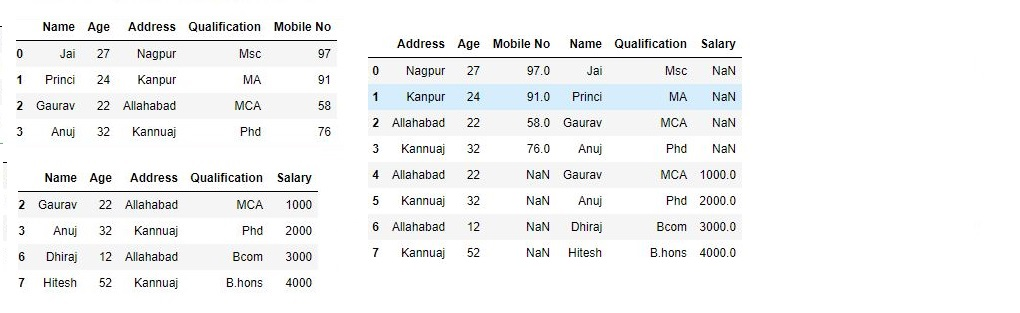Concatenating DataFrame with group keys :
In order to concat dataframe with group keys, we override the column names with the use of the keys argument. Keys argument is to override the column names when creating a new DataFrame based on existing Series.

```
# importing pandas module
import pandas as pd

# Define a dictionary containing employee data
data1 = {'Name':['Jai', 'Princi', 'Gaurav', 'Anuj'],
'Age':[27, 24, 22, 32],
'Qualification':['Msc', 'MA', 'MCA', 'Phd']}

# Define a dictionary containing employee data
data2 = {'Name':['Abhi', 'Ayushi', 'Dhiraj', 'Hitesh'],
'Age':[17, 14, 12, 52],
'Qualification':['Btech', 'B.A', 'Bcom', 'B.hons']}

# Convert the dictionary into DataFrame
df = pd.DataFrame(data1,index=[0, 1, 2, 3])

# Convert the dictionary into DataFrame
df1 = pd.DataFrame(data2, index=[4, 5, 6, 7])

print(df, "\n\n", df1)

```

Now we use keys as an argument.

```# using keys
frames = [df, df1 ]

res = pd.concat(frames, keys=['x', 'y'])
res
```

Output :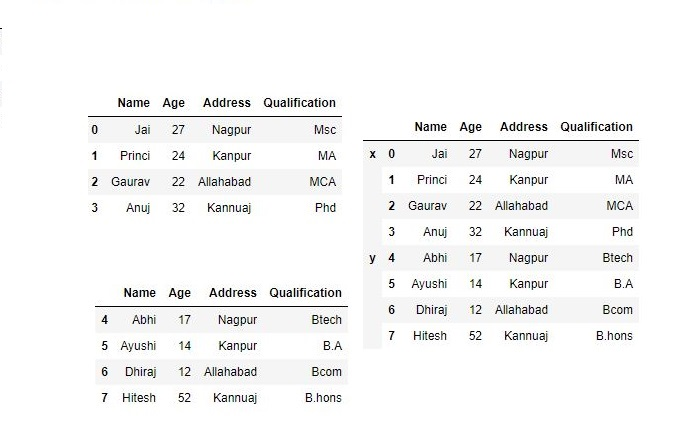Concatenating with mixed ndims :
User can concatenate a mix of Series and DataFrame. The Series will be transformed to DataFrame with the column name as the name of the Series.

```# importing pandas module
import pandas as pd

# Define a dictionary containing employee data
data1 = {'Name':['Jai', 'Princi', 'Gaurav', 'Anuj'],
'Age':[27, 24, 22, 32],
'Qualification':['Msc', 'MA', 'MCA', 'Phd']}

# Convert the dictionary into DataFrame
df = pd.DataFrame(data1,index=[0, 1, 2, 3])

# creating a series
s1 = pd.Series([1000, 2000, 3000, 4000], name='Salary')

print(df, "\n\n", s1)
```

Now we are going to mix Series and dataframe together

```# combining series and dataframe
res = pd.concat([df, s1], axis=1)

res
```

Output :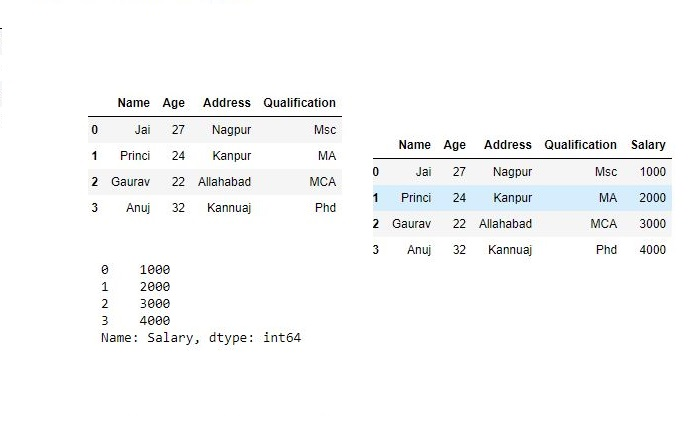#### Merging DataFrame

Pandas have options for high-performance in-memory merging and joining. When we need to combine very large DataFrames, joins serve as a powerful way to perform these operations swiftly. Joins can only be done on two DataFrames at a time, denoted as left and right tables. The key is the common column that the two DataFrames will be joined on. It’s a good practice to use keys which have unique values throughout the column to avoid unintended duplication of row values. Pandas provide a single function, merge(), as the entry point for all standard database join operations between DataFrame objects.
There are four basic ways to handle the join (inner, left, right, and outer), depending on which rows must retain their data.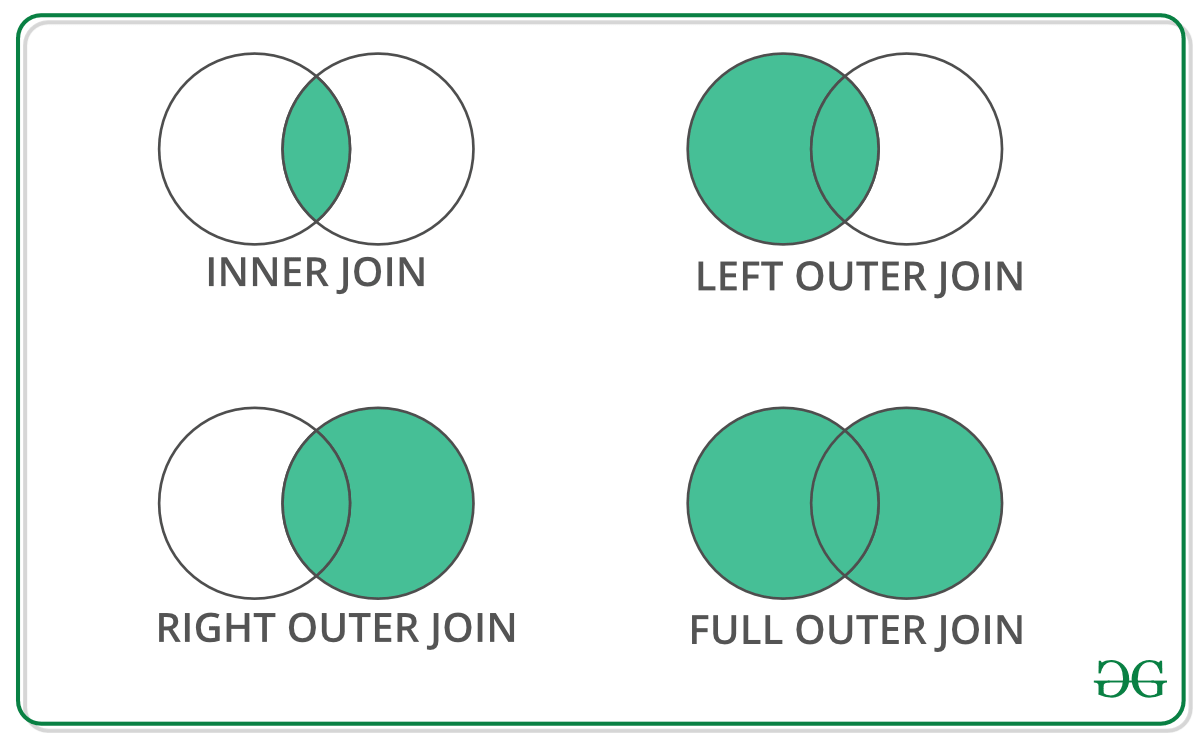Code #1 : Merging a dataframe with one unique key combination

```# importing pandas module
import pandas as pd

# Define a dictionary containing employee data
data1 = {'key': ['K0', 'K1', 'K2', 'K3'],
'Name':['Jai', 'Princi', 'Gaurav', 'Anuj'],
'Age':[27, 24, 22, 32],}

# Define a dictionary containing employee data
data2 = {'key': ['K0', 'K1', 'K2', 'K3'],
'Qualification':['Btech', 'B.A', 'Bcom', 'B.hons']}

# Convert the dictionary into DataFrame
df = pd.DataFrame(data1)

# Convert the dictionary into DataFrame
df1 = pd.DataFrame(data2)

print(df, "\n\n", df1)

```

Now we are using `.merge()` with one unique key combination

```# using .merge() function
res = pd.merge(df, df1, on='key')

res
```

Output :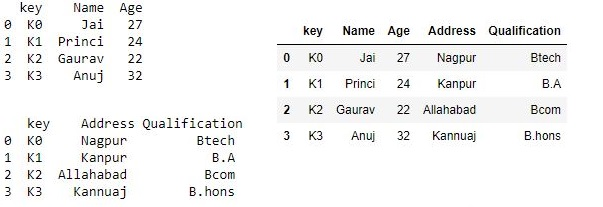Code #2: Merging dataframe using multiple join keys.

```# importing pandas module
import pandas as pd

# Define a dictionary containing employee data
data1 = {'key': ['K0', 'K1', 'K2', 'K3'],
'key1': ['K0', 'K1', 'K0', 'K1'],
'Name':['Jai', 'Princi', 'Gaurav', 'Anuj'],
'Age':[27, 24, 22, 32],}

# Define a dictionary containing employee data
data2 = {'key': ['K0', 'K1', 'K2', 'K3'],
'key1': ['K0', 'K0', 'K0', 'K0'],
'Qualification':['Btech', 'B.A', 'Bcom', 'B.hons']}

# Convert the dictionary into DataFrame
df = pd.DataFrame(data1)

# Convert the dictionary into DataFrame
df1 = pd.DataFrame(data2)

print(df, "\n\n", df1)

```

Now we merge dataframe using multiple keys

```# merging dataframe using multiple keys
res1 = pd.merge(df, df1, on=['key', 'key1'])

res1

```

Output :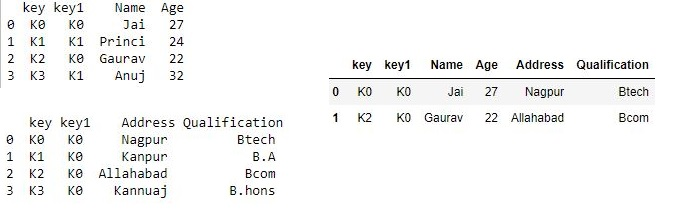Merging dataframe using `how` in an argument:
We use how argument to merge specifies how to determine which keys are to be included in the resulting table. If a key combination does not appear in either the left or right tables, the values in the joined table will be NA. Here is a summary of the how options and their SQL equivalent names:

Merge method Join Name Description
```# importing pandas module
import pandas as pd

# Define a dictionary containing employee data
data1 = {'key': ['K0', 'K1', 'K2', 'K3'],
'key1': ['K0', 'K1', 'K0', 'K1'],
'Name':['Jai', 'Princi', 'Gaurav', 'Anuj'],
'Age':[27, 24, 22, 32],}

# Define a dictionary containing employee data
data2 = {'key': ['K0', 'K1', 'K2', 'K3'],
'key1': ['K0', 'K0', 'K0', 'K0'],
'Qualification':['Btech', 'B.A', 'Bcom', 'B.hons']}

# Convert the dictionary into DataFrame
df = pd.DataFrame(data1)

# Convert the dictionary into DataFrame
df1 = pd.DataFrame(data2)

print(df, "\n\n", df1)

```

Now we set `how = 'left'` in order to use keys from left frame only.

```# using keys from left frame
res = pd.merge(df, df1, how='left', on=['key', 'key1'])

res
```

Output :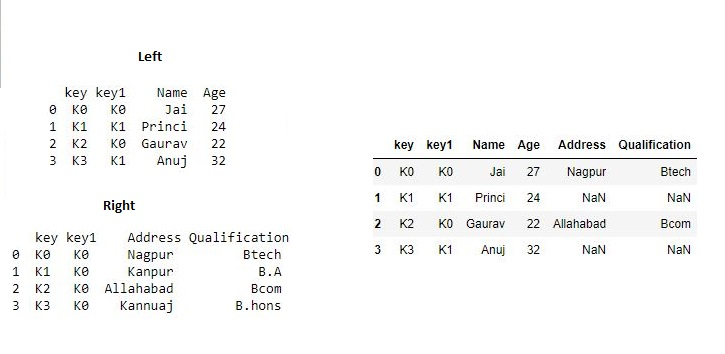Now we set `how = 'right'` in order to use keys from right frame only.

```# using keys from right frame
res1 = pd.merge(df, df1, how='right', on=['key', 'key1'])

res1
```

Output :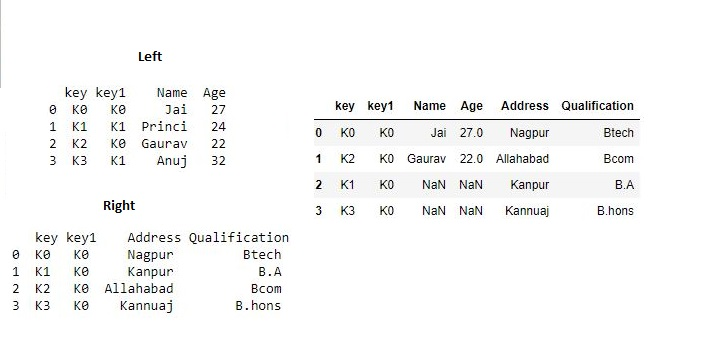Now we set `how = 'outer'` in order to get union of keys from dataframes.

```# getting union  of keys
res2 = pd.merge(df, df1, how='outer', on=['key', 'key1'])

res2
```

Output :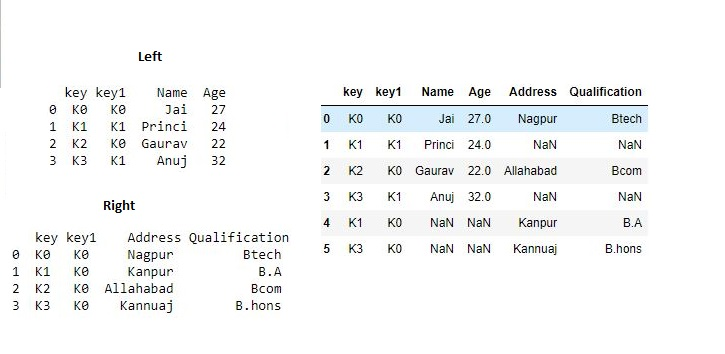Now we set `how = 'inner'` in order to get intersection of keys from dataframes.

```# getting intersection of keys
res3 = pd.merge(df, df1, how='inner', on=['key', 'key1'])

res3
```

Output :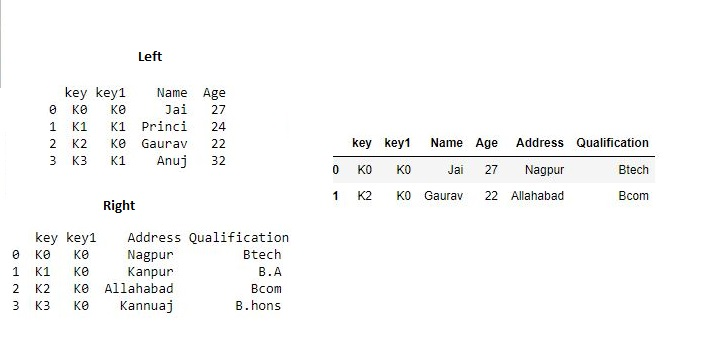#### Joining DataFrame

In order to join dataframe, we use `.join()` function this function is used for combining the columns of two potentially differently-indexed DataFrames into a single result DataFrame.

```# importing pandas module
import pandas as pd

# Define a dictionary containing employee data
data1 = {'Name':['Jai', 'Princi', 'Gaurav', 'Anuj'],
'Age':[27, 24, 22, 32]}

# Define a dictionary containing employee data
'Qualification':['MCA', 'Phd', 'Bcom', 'B.hons']}

# Convert the dictionary into DataFrame
df = pd.DataFrame(data1,index=['K0', 'K1', 'K2', 'K3'])

# Convert the dictionary into DataFrame
df1 = pd.DataFrame(data2, index=['K0', 'K2', 'K3', 'K4'])

print(df, "\n\n", df1)

```

Now we are use `.join()` method in order to join dataframes

```# joining dataframe
res = df.join(df1)

res
```

Output :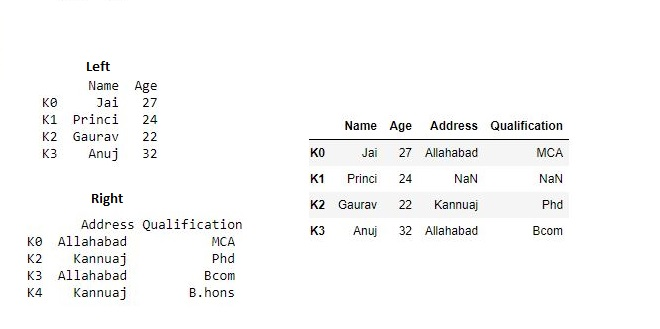Now we use `how = 'outer'` in order to get union

```# getting union
res1 = df.join(df1, how='outer')

res1
```

Output :Joining dataframe using `on` in an argument :
In order to join dataframes we use `on` in an argument. `join()` takes an optional on argument which may be a column or multiple column names, which specifies that the passed DataFrame is to be aligned on that column in the DataFrame.

```# importing pandas module
import pandas as pd

# Define a dictionary containing employee data
data1 = {'Name':['Jai', 'Princi', 'Gaurav', 'Anuj'],
'Age':[27, 24, 22, 32],
'Key':['K0', 'K1', 'K2', 'K3']}

# Define a dictionary containing employee data
'Qualification':['MCA', 'Phd', 'Bcom', 'B.hons']}

# Convert the dictionary into DataFrame
df = pd.DataFrame(data1)

# Convert the dictionary into DataFrame
df1 = pd.DataFrame(data2, index=['K0', 'K2', 'K3', 'K4'])

print(df, "\n\n", df1)

```

Now we are using `.join` with “on” argument

```# using on argument in join
res2 = df.join(df1, on='Key')

res2
```

Output :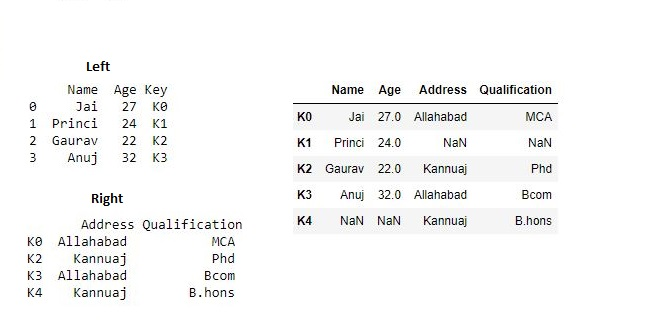Joining singly-indexed DataFrame with multi-indexed DataFrame :
In order to join singly indexed dataframe with multi-indexed dataframe, the level will match on the name of the index of the singly-indexed frame against a level name of the multi-indexed frame.

```# importing pandas module
import pandas as pd

# Define a dictionary containing employee data
data1 = {'Name':['Jai', 'Princi', 'Gaurav'],
'Age':[27, 24, 22]}

# Define a dictionary containing employee data
'Qualification':['MCA', 'Phd', 'Bcom', 'B.hons']}

# Convert the dictionary into DataFrame
df = pd.DataFrame(data1, index=pd.Index(['K0', 'K1', 'K2'], name='key'))

index = pd.MultiIndex.from_tuples([('K0', 'Y0'), ('K1', 'Y1'),
('K2', 'Y2'), ('K2', 'Y3')],
names=['key', 'Y'])

# Convert the dictionary into DataFrame
df1 = pd.DataFrame(data2, index= index)

print(df, "\n\n", df1)

```

Now we join singly indexed dataframe with multi-indexed dataframe

```# joining singly indexed with
# multi indexed
result = df.join(df1, how='inner')

result
```

Output :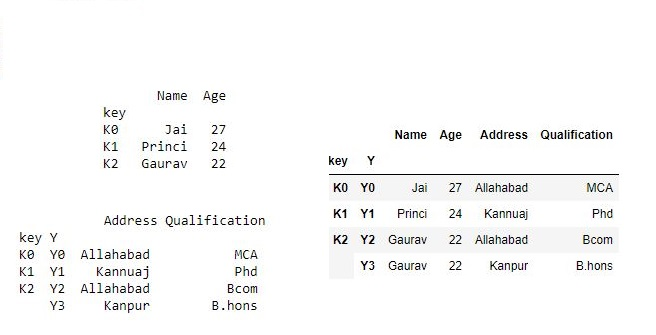My Personal Notes arrow_drop_up